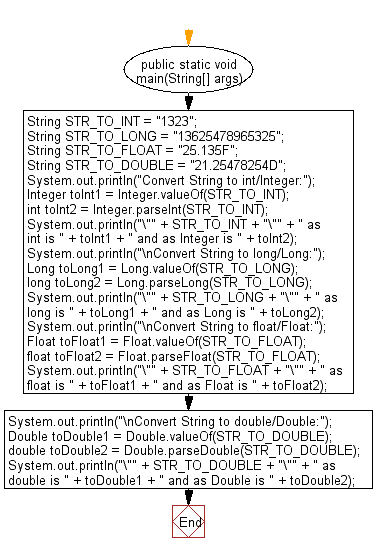﻿ Java exercises: Convert a given String to int, long, float and double - w3resource# Java String Exercises: Convert a given String to int, long, float and double

## Java String: Exercise-102 with Solution

Write a Java program to convert a given String to int, long, float and double.

Sample Solution:

Java Code:

``````public class Main {
public static void main(String[] args) {
String STR_TO_INT = "1323";
String STR_TO_LONG = "13625478965325";
String STR_TO_FLOAT = "25.135F";
String STR_TO_DOUBLE = "21.25478254D";

System.out.println("Convert String to int/Integer:");
Integer toInt1 = Integer.valueOf(STR_TO_INT);
int toInt2 = Integer.parseInt(STR_TO_INT);
System.out.println("\"" + STR_TO_INT + "\"" + " as int is "
+ toInt1 + " and as Integer is " + toInt2);

System.out.println("\nConvert String to long/Long:");
Long toLong1 = Long.valueOf(STR_TO_LONG);
long toLong2 = Long.parseLong(STR_TO_LONG);
System.out.println("\"" + STR_TO_LONG + "\"" + " as long is "
+ toLong1 + " and as Long is " + toLong2);
System.out.println("\nConvert String to float/Float:");
Float toFloat1 = Float.valueOf(STR_TO_FLOAT);
float toFloat2 = Float.parseFloat(STR_TO_FLOAT);
System.out.println("\"" + STR_TO_FLOAT + "\"" + " as float is "
+ toFloat1 + " and as Float is " + toFloat2);

System.out.println("\nConvert String to double/Double:");
Double toDouble1 = Double.valueOf(STR_TO_DOUBLE);
double toDouble2 = Double.parseDouble(STR_TO_DOUBLE);
System.out.println("\"" + STR_TO_DOUBLE + "\"" + " as double is "
+ toDouble1 + " and as Double is " + toDouble2);
}
}
``````

Sample Output:

```Convert String to int/Integer:
"1323" as int is 1323 and as Integer is 1323

Convert String to long/Long:
"13625478965325" as long is 13625478965325 and as Long is 13625478965325

Convert String to float/Float:
"25.135F" as float is 25.135 and as Float is 25.135

Convert String to double/Double:
"21.25478254D" as double is 21.25478254 and as Double is 21.25478254
false
```

Pictorial Presentation:Flowchart:Java Code Editor:

Improve this sample solution and post your code through Disqus

What is the difficulty level of this exercise?

﻿

## Java: Tips of the Day

Parsing dates:

```import java.io.*;
import java.util.*;
import java.text.*;

String s = "2001/09/23 14:39";

SimpleDateFormat formatter = new SimpleDateFormat ("yyyy/MM/dd H:mm");
Date d = formatter.parse(s, new ParsePosition(0));
```